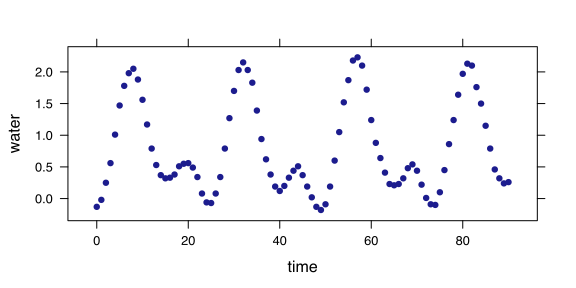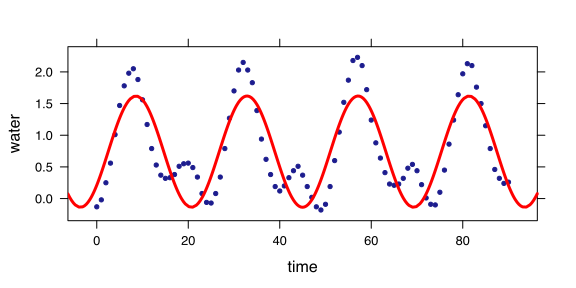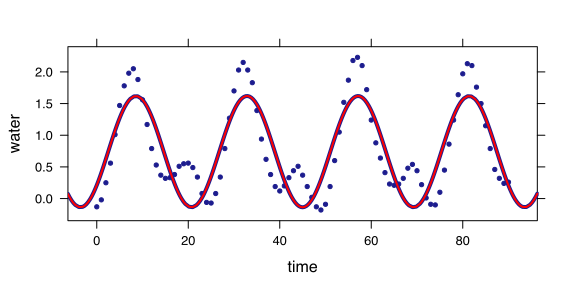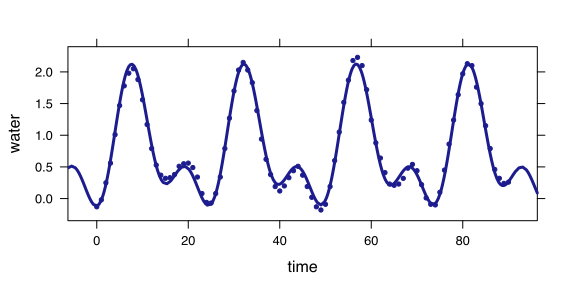# Modeling Tides

The file hawaii.csv contains hour-by-hour measurements of water level in Pearl Harbor, Hawaii, over approximately 4 days.

tides = fetchData("hawaii.csv")
xyplot(water ~ time, data = tides)How to model these data? They are repetitive, which suggests a sine-wave model. We need to know the period, amplitude, phase, and offset. Looking at the graph suggests a period of about 24 hours, as seems sensible.

f0 = fitModel(water ~ A + B * sin(2 * pi * (time - T0)/P), data = tides, start = list(P = 24))
xyplot(water ~ time, data = tides, pch = 20)
plotFun(f0(time) ~ time, add = TRUE, lwd = 3, col = "red")Here, T0 is the phase of the sine function.

Another way to address the phase issue is to add a cosine of the same period. Fitting that sort of model, and comparing to the time-shift parameterization shows that they are effectively the same.

f1 = fitModel(water ~ A + B * sin(2 * pi * time/P) + C * cos(2 * pi * time/P),
data = tides, start = list(P = 24))
xyplot(water ~ time, data = tides, pch = 20)
plotFun(f1(time) ~ time, add = TRUE, lwd = 4)
plotFun(f0(time) ~ time, add = TRUE, col = "red")According to the model, what's the period?

That's a pretty rough model. Perhaps that's because there are often two tides per day. So there's something going on with a period of about 12 hours. A new model:

f2 = fitModel(water ~ A + B * sin(2 * pi * time/P1) + C * cos(2 * pi * time/P1) +
D * sin(2 * pi * time/P2) + E * cos(2 * pi * time/P2), data = tides, start = list(P1 = 24,
P2 = 12))
xyplot(water ~ time, data = tides, pch = 20)
plotFun(f2(time) ~ time, add = TRUE, lwd = 3)Much better!

## Questions

1. What's the relationship between the two periods?
2. Do you get a much better model with three or more periods? What should those new periods be?
3. The model is quite good, yet the fundamental period is not 24 hours. Why not?